<img src="//bat.bing.com/action/0?ti=5587743&amp;Ver=2" height="0" width="0" style="display:none; visibility: hidden;">

## Does Your Data Pass Muster?

You got your results and data, but is it accurate? Is it precise? Are your eyes starting to cross from staring at numbers? You aren't alone! This blog will walk you through what you need to do to ensure that the data you are reporting is accurate, precise, and repeatable. (Plus, there is a handy cheat sheet!)

## Analyzing Data

Analytical tests are performed in a multitude of applications for a variety of reasons. The test may be one part of a larger system that ensures process efficacy, product quality and/or that state and federal guidelines are met. Though the reasons may vary, the desired outcome is the same: obtain measurement data. However, the data alone are meaningless without statistical interpretation. Collecting data is only the first part; the data must also be analyzed according to predetermined criteria to establish significance. If you’re not quite sure what I mean by that and I have inadvertently triggered a stress response, I’d like you to first take some deep, calming breaths and then put on your thinking cap - I’m about to walk you through some common statistical analyses used in the practice of analyzing data. I would like to note, however, that data verification practices can be quite complex, and can significantly vary based on the application, analytical test, and process significance. The following information should therefore only be used as a general guideline or introduction to such practices, and should not act as or replace testing verification protocols. If after reading through the following material you are concerned your current process is lacking, fear not! There are specific institutions, contractors, and consultants who specialize in the development and/or assessment of such protocols that are able to assist.

1. How do I summarize data?
• Sample Size, or Replicates
• Mean, or Average
• Precision
• Accuracy
2. How do I know I can trust the data?
• Precision
• Accuracy

## How Do I Summarize Data?

### Sample Size, or Replicates

The first consideration to be made when collecting data is the number of times the same test is performed on the same material, known as sample size, n or the number of replicates. Different applications and testing procedures will have different requirements, where in one application, a sample may be analyzed only once and yet another may require two (duplicate) or three (triplicate) replicates. For example, in an environmental testing laboratory, a multitude of samples are received every day that often require more than one analytical test. By analyzing samples once per test, and grouping a run of samples with specific quality control tests to validate the sample run, resources are maximized and sample is conserved for other tests. Yet in other instances, more than three replicates may be performed, such as when developing new methods and/or determining method performance criteria for new or updated instrumentation. When n ≥ 2 or, when a test is performed, at minimum, in duplicate, the data is often referred to as a data set. Based on your testing protocol and resources, as well as the measurement importance, varying replicates may be utilized.
Now, before we go any further, we must first touch on an aspect commonly applied to statistical terminology. When certain terminology is used, you will notice that it is either expressed as an infinite (immeasurable) or finite (measurable) number. Essentially, this means statisticians may reference an immeasurable statistic when defining a measurable one. Still confused? No worries! We’ll elaborate with the next term we’ll be discussing: mean.

### Average or Mean

When n ≥ 2, the mean or, average can be calculated. The actual population mean, μ or, the “true mean” is based on an infinite number of measurements. So, μ would be the immeasurable reference point, meaning due to real life limitations, such as time and resources, μ cannot actually be determined. It is therefore represented by the measurable sample mean, , which is defined as:
ASTM International (2020), “Sum of the observed values in the sample divided by the sample size” (ASTM E456 Standard Terminology Relating to Quality and Statistics, p. 5).
As the sample size increases, will more accurately represent μ. Let’s look at an example. A data set was collected in triplicate (n = 3) and is presented in Table 1. When calculated in Equation (1), (an estimate of μ) would equal 13.19%.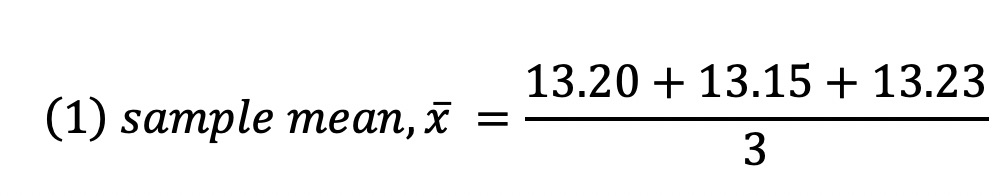Replicate Result (% by vol.) 1 13.20 2 13.15 3 13.23

In addition to summarizing data, the sample mean is also applied to other statistical analyses that may also be used to summarize a data set.
Such analyses that we will be discussing here are also directly related to perhaps the most common consideration pertaining to a data set: “How do I know I can trust this data?” This question is not only valid, but extremely important! After all, insignificant test results are perhaps more unhelpful and detrimental than no result at all. What do I mean by that? Here’s another way to think about it. An analytical test is performed that monitors a product process and most recently, the test reports a result above or below the “ideal” or “expected” range for that part in the process. Now what do you do? Do you assume the results are correct and make adjustments to the process so that it is within specification? The answer is to always limit as much assumption as possible and to make a decision based on data from a trustworthy testing process. So, how do we limit assumption and determine if the test result is trustworthy? Accuracy and precision, of course!

### Accuracy Vs. Precision

The common example of defining accuracy and precision is that of a dart board. As you can see in Figure 1, test results can be one of three combinations in reference to accuracy and precision, where precision is defined as “the closeness of agreement between independent test results obtained under stipulated conditions” and accuracy as “the closeness of agreement between a test result and an accepted reference value” (ASTM, 2020). If results in a data set do not significantly vary then the data has a high level of precision or low level of imprecision, as shown in the dart boards labeled Precise but not accurate and Both accurate and precise. If results do not significantly vary from the known “true value” or, accepted reference value (more on this later) then the data has a high level of accuracy or low level of inaccuracy, as shown in the dart board labeled Both accurate and precise. You may be thinking to yourself, “Hey! Where’s the fourth dart board labeled Accurate but not precise!”, which you may or may not have seen before. Well, it is very unlikely to have high accuracy without high precision, as a value is unlikely to be accurate in the midst of imprecise data. Basically, high precision is required for high accuracy, but high precision alone does not guarantee high accuracy. This is why a testing operation must consider both when analyzing a data set, method criteria, and/or their lab technique.

Figure 1. Accuracy vs. Precision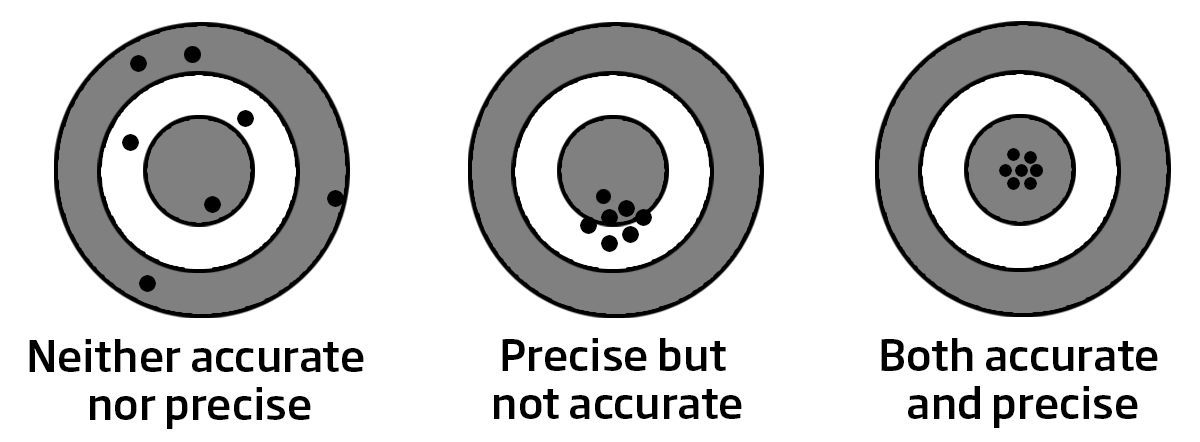### So, how do we determine how precise and accurate a result is? Let’s begin with precision.

Measurements of precision are expressed in terms of imprecision. The most common metric for measuring imprecision is repeatability, where data collection occurs under stipulated conditions. Meaning, before we can discuss how to determine repeatability, we must first discuss how to collect data to determine repeatability.

Imprecision stems from random error, where variation in results may occur from undeterminable aspects such as operator, equipment, lab environment, etc., so to minimize these variations, the stipulated conditions for data collection must be followed. To test for repeatability, data collection must occur under repeatability conditions:

ASTM International (2020), “conditions where independent test results are obtained with the same method on identical test items in the same laboratory by the same operator using the same equipment within short intervals of time” (ASTM E456 Standard Terminology Relating to Quality and Statistics, p. 7).

So, if determining imprecision as repeatability, data must be collected:
• On the same source material
• In the same laboratory
• By the same operator
• Using the same equipment
• Within a small amount of time, as can be reasonably done

## How Do I Know I Can Trust The Data?### Now that you have collected data under the stipulated repeatability conditions, we are ready to determine repeatability.

Repeatability is most commonly presented as standard deviation. When analyzing imprecision, we are determining how far from the mean a group of results fall; the higher the deviation from the mean, the higher the imprecision. Just like we touched on when describing the mean, standard deviation can also be expressed based on an infinite (immeasurable) or finite (measurable) number; σ denotes the variance of an infinite set of experimental data and s, the estimated standard deviation, denotes the variance of a finite set of experimental data (Equation(2)).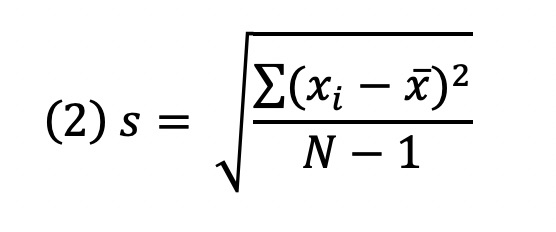Standard deviation may also be published as relative standard deviation (rsd)  or percent relative standard deviation (% rsd). When s is divided by , the deviation is expressed as a fraction of the mean (rsd) and can then be converted to a percentage by multiplying by 100 (% rsd) (3).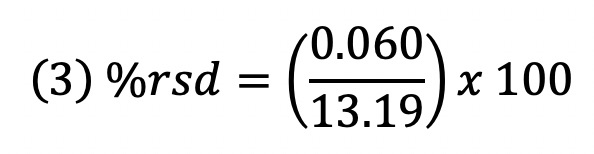Let's refer back to the data set presented in Table 1, and summarize what we know so far in Table 2, where in order for s and the % rsd to be truly representative of repeatability, the data was collected under repeatability conditions

 Replicate Result (% by vol.) 1 13.20 2 13.15 3 13.23 x̅ 13.19 s 0.040 % rsd 0.31

### So, you’ve collected your data under repeatability conditions and calculated the repeatability. Now what?

Well, here is when your predefined testing criteria come into action. Let’s return to our environmental testing laboratory example. It turns out that one of the lab’s quality control tests involved analyzing one of the samples in duplicate so that a variation between those two tests could be calculated. The technician then refers to the specified precision metric for that test, dictated as a maximum allowable deviation, to ensure compliance for precision for the entire sample run. If you do not have a variance protocol and are not sure where to begin, reference methods are a great place to start. Reference methods or, standard methods are published by institutions like AOAC, ASTM, and ISO, and may provide statistical guidelines, such as variance. Such guidelines are most often based on interlaboratory studies, where a specified number of participating laboratories analyze the same, or similar materials via the specified procedure, and the resulting data then analyzed by the publishing method institute to establish statistical guidelines for the test. Sometimes, based on the test, the guidelines may also be differentiated based on sample type and/or concentration. In either case, it’s important the allowable deviation is chosen accordingly and is not too liberal or too conservative to avoid “passing” measurement results with higher variation than is normal to the test method or “rejecting” those that demonstrate normal variation to the test method. Furthermore, because there are more ways of expressing imprecision than discussed here, it’s important that the calculated imprecision metric is the same as the one used in the guideline.

### Let’s move on to the next factor in trustworthy data: accuracy.

When analyzing precision, the “true value” of the material to-be-tested does not need to be known since we are determining how close the results are to the calculated mean. Therefore, a data set from tests performed on a sample or reference material can be analyzed for precision, where, in contrast, accuracy can only be determined on a reference material that has an accepted reference value, “a value that serves as an agreed-upon reference for comparison” (ASTM, 2020). There are varying types of reference materials available – certified reference materials, calibration standards, quality control materials, check standards– with varying degrees of purity, certainty, sample similarity, and stability.

.(1) Purity – varying types of materials that are not measured (also known as impurities) may be present, such as water, metals, alcohols, etc., and provided on the bottle/container or in a certificate of analysis.
(2) Certainty – the level of uncertainty of the accepted reference value; usually displayed as a +/- in a certificate of analysis.
(3) Sample similarity – the testing material may be available in a matrix similar to the sample to-be-tested, i.e. edible oil.
(4) Stability – the reliability of the accepted reference value will vary based on certain characteristics of the standard, such as the state in which it was prepared and preserved.

The significance of the reference material type is based on the overall objective. For instance, will it be used to develop trends over time, where product stability will be important or as part of the validation procedure on a new product formulation, where its likeness to the actual product to-be-tested is of central concern? Based on the objective of the test, a specific reference material may or may not be sufficient, and based on the testing protocol, more than one type of reference material may be used at the same or at different stages of the testing process. Nevertheless, the common theme in all reference materials is that there is an accepted reference value.

Like precision, accuracy is tested in terms of inaccuracy and the most common way of specifying inaccuracy is by bias:

ASTM International (2020), “The difference between the expectation of a test result and an accepted reference value” (ASTM E 456 Standard Terminology Relating to Quality and Statistics, p. 2).

So, you’ve chosen your reference material and collected data following the test procedure. Without any type of data analysis, you notice right away that the test result differs from the reference value. Variation is expected, as actual science is not perfect and will vary from the theoretical. The important aspects in regards to inaccuracy are the amount and direction of variance from the accepted reference value. Let’s return to the data set from the previous Tables to see what I mean by that. Let’s say QC Sample #A1 specifies a reference value of 13.01% +/-0.26. We then reference Equation (4) and determine the variation of  (13.19%) from the reference value (13.01), as shown in Equation (5):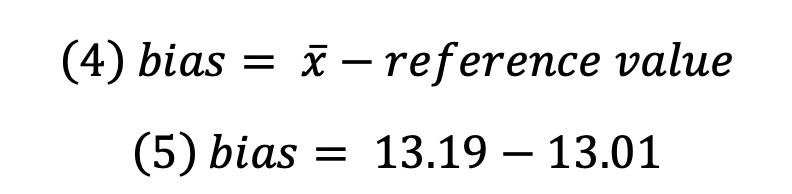As we can see, there is a variation of +0.18 from the reference value; as mentioned above, both the degree and direction of inaccuracy is important, where a positive (+) value dictates a positive bias. Figure 2 displays a positive bias.

Figure 2. Depiction of both bias and precision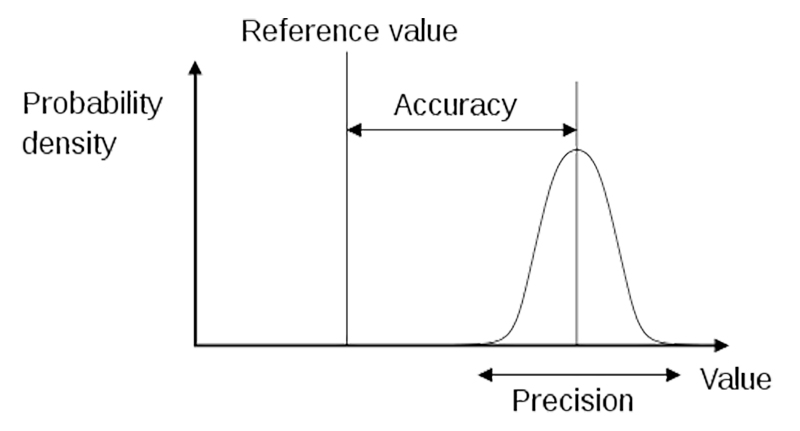AOAC Appendix F: Guidelines for Standard Method Performance Requirements (2016)

### Our results are biased? Uh oh! Right?Well, no, not necessarily. One way of determining if the level of bias is out of specification is by the level of uncertainty of the reference value. But, wait, I thought the purpose of the reference material was that the reference value is known? Even a reference value cannot be known with absolute certainty and, based on the reference material utilized, the level of uncertainty may be provided. As we can see from the provided specifications, usually provided in a Certificate of Analysis, our results fell within the level of uncertainty for the reference material. This means that if we formatted the specification into words, it would state: the “true value” falls somewhere within +/-0.26 of 13.01% or, more specifically, somewhere within 12.75 and 13.27%. The sample mean for the data set was 13.19%, so we fell within those specified limits. Based on the testing lab, however, the internal allowable limits may be more or less strict.

Another way to look at or visualize variation from the accepted reference value is by percent recovery of the material we are testing for, where how much was recovered (measured value) is compared to how much theoretically should have been recovered (the reference value). Simply put, we would divide our measured value by our reference value and multiply by 100, as shown in Equation (6).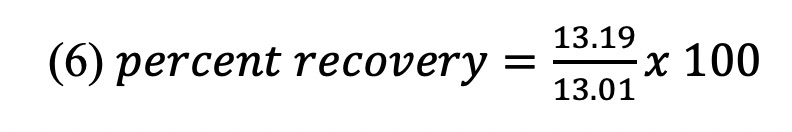This then yields a recovery of 101.4%. This also dictates a positive bias, as we recovered more than the specified reference value. If there was a negative bias, then the recovery would equal less than 100%.

But, hang on, how can more than 100% of anything be measured? Well, it can’t. Bias and percent recovery demonstrate one or more systematic error(s) somewhere in our testing process that is accounting for more material than what the reference value states is there. Whether or not this is actually a problem is – you guessed it – based on your pre-determined testing criteria, as well as the trend for this test (more on that in just a bit). Let’s circle back to the case of the environmental testing lab to see how they analyze inaccuracy. Another routine quality control test that they perform is called a matrix spike, where a sample is “spiked” or, dosed with a precise and known amount of material of interest. Since the amount of material that was added is accurately known, a recovery calculation can be performed to ensure a certain percentage was recovered during the test run. If the matrix spike falls within the acceptable recovery limits then it is assumed the results for the non-spiked samples are also accurate.

Overtime, these positive or negative recoveries or biases can be used to develop trends. Testing for inaccuracy is not always as straight forward as a single calculation metric. In order to develop the trend of the test method results, the direction and degree of bias is analyzed over time on the same sample, usually by use of control charts. This means in order to utilize a control chart to determine trends, a singular data set on a reference material is nowhere near enough data to determine the normal direction and degree of bias. Control charts are great predictors of when one or more aspects of the testing process is slowly or quickly degrading. If you always know where you stand for a specific test, results wise, it is easier to determine when results are “off” or trending away from what is normal for your lab.

If you’ve just read this information and still don’t know where your data stands in relation to where it should stand, a great place to start is to research proficiency testing (PT) for the test of interest. PT is a way of evaluating your performance as a lab against other laboratories. When you participate in a PT, a standard method institution or an affiliated laboratory provides your lab with a reference material to-be-tested by your laboratory as you normally would a sample. Afterwards, you obtain a report stating where your results fell in relation to results from other unidentified participating labs, establishing your level of competence in that specific test area and indicating if certain measures need to be taken to perfect your testing process.

Let’s review what we discussed in the blog. We have provided the summary in a helpful, downloadable PDF, as well.

## Summarizing Data

### A Cheat Sheet for Terms, Knowledge, & Facts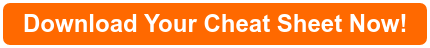SUMMARIZING DATA The number of times the same sample is analyzed per tests is called the sample size (n). This may also be labeled as replicates. When 3 or more replicates are performed (n ≥ 2), results can be further analyzed. Further Analyses Definition(s) 1. Mean (x̅) The  sum of all results divided by the sample size. 2. Precision a. The closeness of agreement between test results obtained under stipulated conditions; b. Can be measured on a sample/standard. 3. Accuracy a. The closeness of agreement between test results and an accepted reference value; b. Can only be measured on a reference material with an accepted reference value.AOAC Appendix F: Guidelines for Standard Method Performance Requirements (2016)

 PRECISION The level of imprecision is often measured as repeatability, where measurements must be performed under repeatability conditions: 1. On the same source material. 2. In the same laboratory. 3. By the same operator. 4. Using the same equipment. 5. Within a small amount of time, as can be reasonably be done. Repeatability is often calculated as the standard deviation (s) or percent relative standard deviation (% rsd). 1. Both dictate the consistency of measurement data by calculating the variation of the results from the calculated mean. 2. As the deviation between results increase, both s and % rsd also increase. 3. Testing laboratories generally have an acceptable deviation per analytical test. 4. Standard methods may provide guidelines for precision.

 ACCURACY Determined with a reference material, which has an accepted reference value. A variety of different reference materials exist and may differ based on: 1. Purity2. Certainty3. Sample similarity4. Stability Inaccuracy can be calculated as bias or percent recovery, where the amount and direction of deviation from the measured value to the accepted reference value is determined. a. Positive bias or greater than 100% recovery – the measured value is greater than the accepted reference value b. Negative bias or less than 100% recovery – the measured value is less than the accepted reference value Testing laboratories generally have an acceptable +/- from a specified reference material. Control charts can be utilized to develop trends over time based on bias. ADDITIONAL TOOLS Proficiency testing for a specific test establishes where your laboratory’s testing abilities fall in comparison to others who are also performing the same test. Specific companies and consultants are available to further assist in establishing or auditing current testing protocols.